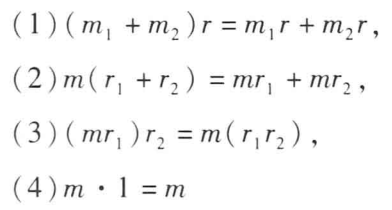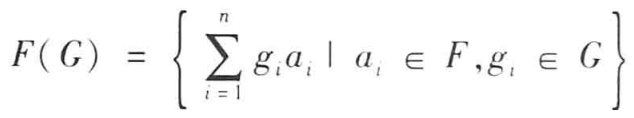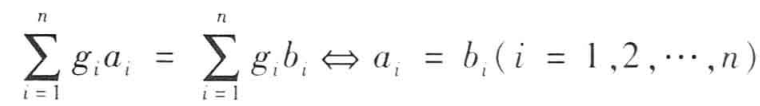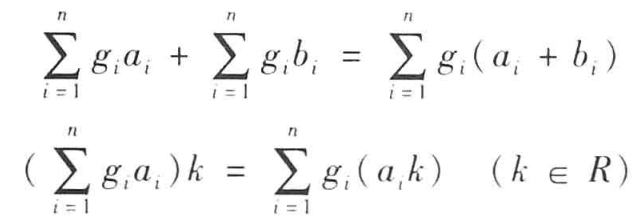• Q中一网友贡献一个模板   C++语言: #include #include #include #include #define DLEN 4   //一个整数代表四位 #define MAXN 9999  //等于 10^DLEN - 1 //特别说明：只进行减时可以改为一个整数...

Q群中一网友贡献的一个模板

C++语言:

#include
<cstring>#include
<cstdio>#include
<iostream>#include
<iomanip>#define DLEN 4

//一个整数代表四位#define MAXN 9999
//等于 10^DLEN - 1//特别说明：只进行加减时可以改为一个整数代表9位#define MAXSIZE 100
//用来表示大整数的数组的长度//依赖头文件:
<cstring>class
bigint
{

//大整数类public:
int a[MAXSIZE];
int len;
bigint() {len = 1; memset(a,0,sizeof(a));}
bigint(const int);

bigint(const char*);
bigint(const bigint &);
bigint &operator=(const
bigint &);
operator
int();};bigint::bigint(const int b)
{
int c,d = b;
len = 0;
memset(a,0,sizeof(a));
while (d > MAXN) {

c
= d - (d / (MAXN + 1)) * (MAXN + 1);

d
= d / (MAXN + 1);

a[len++] = c;
}
a[len++] = d;}bigint::bigint(const char *s) {//字符串大整数的格式为左端最高位
int t,k,index,l,i,j;
memset(a,0,sizeof(a));
l = strlen(s) - 1;
len = l++ / DLEN + 1;
index = 0;
for(i = l - 1; i >= 0; i -= DLEN) {

t
= 0;

k
= i - DLEN + 1 < 0 ? 0 : i - DLEN + 1;

for (j = k; j <= i; j++)

t
= t * 10 + s[j] - '0';

a[index++] = t;
}}bigint::bigint(const bigint & T) {
*this = T;}bigint & bigint::operator=(const
bigint & n) {
memcpy(a, n.a, sizeof(a));

len
= n.len;
return *this;}bigint::operator
int()
{
int i,n = 0;
for (i = len - 1; i >= 0; i--) {

n *=
MAXN+1;

n +=
a[i];
}
return n;}//需使用头文件:
<cstdio>void
bigintscan(bigint * b) {
char ch[MAXSIZE*DLEN];
scanf("%s",ch);
*b = ch;
return ;}void
bigintprint(bigint &b) {
int i;
printf("%d",b.a[b.len - 1]);
for(i = b.len - 2 ; i >= 0 ; i--) {

printf("d",b.a[i]); //修改DLEN时应同时修改此处
}
return;}//需使用头文件：
<iostream>
<iomanip>std::istream& operator>>(std::istream
&
in,  bigint & b) {
char ch[MAXSIZE*DLEN];
in >>
ch;
b = ch;
return in;}std::ostream& operator<<(std::ostream&
out,  bigint& b) {
int i;
out <<
b.a[b.len - 1];
for (i = b.len - 2; i >= 0; i--) {

out
<<
std::setw(DLEN) <<
std::setfill('0') <<
b.a[i];
}
return out;}//a>T返回1;a<T返回-1;相等返回0int
comp(bigint const & a, bigint const & T) {
int ln;
if (a.len > T.len) return 1;
if (T.len > a.len) return -1;
ln = a.len;
while (ln--) {

if (a.a[ln] != T.a[ln]) break;
}
if (ln == -1) return 0;
if (a.a[ln] > T.a[ln]) return 1;
if (a.a[ln] < T.a[ln]) return -1;}bool
operator>(bigint
&
a, bigint & T) { return
(comp(a,T) == 1); }bool
operator==(bigint
&
a,bigint & T) { return
(comp(a,T) == 0); }bool
operator<(bigint
&
a, bigint & T) { return
(comp(a,T) ==-1); }bool
operator!=(bigint
&
a,bigint & T) { return
(comp(a,T) != 0); }bool
operator>=(bigint
&
a,bigint & T) { return
(comp(a,T) !=-1); }bool
operator<=(bigint
&
a,bigint & T) { return
(comp(a,T) != 1); }bool
operator>(bigint
&
a, int t) { return
(comp(a,bigint(t)) == 1); }bool
operator==(bigint
&
a,int t) { return
(comp(a,bigint(t)) == 0); }bool
operator<(bigint
&
a, int t) { return
(comp(a,bigint(t)) ==-1); }bool
operator!=(bigint
&
a,int t) { return
(comp(a,bigint(t)) != 0); }bool
operator>=(bigint
&
a,int t) { return
(comp(a,bigint(t)) !=-1); }bool
operator<=(bigint
&
a,int t) { return
(comp(a,bigint(t)) != 1); }void
operator+=(bigint
&
a, bigint & T) {
int i,ln;
ln = T.len > a.len ? T.len : a.len;
for (i = 0; i < ln; ++i) {

a.a[i] += T.a[i];

if (a.a[i] > MAXN) {

++a.a[i
+ 1];

a.a[i]
-= MAXN+1;

}
}
a.len = a.a[ln] != 0 ? ln + 1 : ln;
return;}void
operator-=(bigint
&
a, bigint & T) {
for (int i = 0; i < a.len; ++i) {

a.a[i] -= T.a[i];

if (a.a[i] < 0) {

if
(i+1 != a.len) --a.a[i+1];

a.a[i]
+= MAXN+1;

}
}
if (a.a[a.len-1] == 0) a.len--;
return;}void
operator+=(bigint
&a, int n)
{

展开全文• 文章目录1 的定义 1 的定义 可看作线性空间概念的推广 只是把线性空间的基域改为有单位元的环   定义1.1.1 ...MMM是加群,即(M,+)(M,+)(M,+)是可换群 ...上面定义了一种新的运算，用加群里的元素\$\t
文章目录1 模的定义
1 模的定义

可看作线性空间概念的推广
只是把线性空间的基域改为有单位元的环

定义1.1.1

$R$是有单位元的环

$M$是加群,即$(M,+)$是可换群

还有一个“右数乘”运算$M\times R\subseteq M$满足如下性

右数乘，右数乘，右边是个数！也就是环上的数字哦！
1个交换群叫做一个加群
$R$是$M$的啥啊？也就是$M\times R$是属于$M$的哦！
上面定义了一种新的运算，用加群里的元素$\times$环里的元素！$m,m_1,m_2\in M$
$r,r_1,r_2\in R$
$1$是$R$单位元
则称$M$是一个右$R$-模

记为$M_R$或$M=M_R$

即对任意$r\in R$,$m\in M$

$M$中有唯一确定元素与之对应,写为$mr$

类似,如果将“右数乘”$mr$改写为“左数乘”$rm$

就可得到左$R$-模定义

如果$R$是交换环

右$R$-模可看作是左$R$-模
此时只需定义$rm=mr(r\in R,m\in M)$就可,反之亦然

交换环的情况下,右$R$-模与左$R$-模可不加区别
右$R$-模与左$R$-模的理论是平行的,以后无特别说明

所涉及的$R$-模均是右$R$-模

$V$是域$P$上线性空间

则是个右$P$-模

设$R$是一个有单位元的环

$A$是$R$的右理想,则$A$是$R$的一个右$R$-模

$F$是域$E$上的子域,则$E$是一个右$F$-模
$C$是加群

则$G$是一个右$\mathbb{Z}$-模($\mathbb{Z}$是整数环)
因为若$k$是一个整数$x\in G$
按通常倍数定义:易验证它满足模定义的四条件
每个加群都可看作是一个$\mathbb{Z}$-模

通常对加群与$\mathbb{Z}$-模不区别

$F$是域,$G=\{g_1,g_2,…,g_n\}$

其他运算记为乘法
令这里$g_ia_i$是形式定义,没具体定义

$F(G)$中两元素相等,规定为再给$F(G)$定义如下的“加法”与“数乘”易验证,$F(G)$是个以$g_1,g_2,…,g_n$为基的$F$上线性空间

因而$F(G)$是个右$F$-模

下介绍双模的概念,所涉及的模可以是左模,也可以是右模。

定义1.1.2
设$M$是$R$-模又是$S$-模(对这两者,M的加法结构是保持一致的)
如果$M$对$R$的“数乘”

与
对$S$的“数乘”是可交换的,则称$M$是一个$(S,R)$-双模

$M$是右$R$-模又是右$S$-模

则有$(mr)s=(ms)r$
以上是对任意$m∈M,r∈R,s∈S$

由此见
$(S,R)$-双模可分同侧的(同左或同右)与异側的(一个是左模,另一个是右模)两类。
对异侧的,当需要表明侧向时,常以KaTeX parse error: Double subscript at position 4: s_M_̲r

表示$M$为左$S$模且是右$R$-模的双模


展开全文• 在盲化CZKID签名方案时，仅添加了模加运算，而LR98盲签名方案在盲化CS97签名方案时，则添加了求双重离散对数、离散对数根以及随机置换运算。两者比较，新提出方案计算复杂度更低，效率更高。信息安全
• 该方案建立在标准模型下,通过对混合阶双线性运算的正交性和向量属性运用,成就了短尺寸固定密文,具有较高计算效率与存储效率.该方案安全性依赖于3个简单静态假设,安全性证明显示该方案达到了完全安全性...
• RSA算法实现 摘 要 本文设计是一套完整实用RSA文件加密解决方案并具体编码实现本文采用费马小定理测试素数使用Montgomery加快大数运算用C++实现RSA加密算法类库并在32位windows平台封装成组件在.Net平台...
• 第7章分别就、环、域和等抽象代数基本概念进行梳理分析;第8章主要介绍了椭圆曲线相关性质。这样把包括三个数学难解问题在内、面向单钥制和双钥制加密及相关认证技术数学基础知识进行了完整梳理，构成...
• 在信息安全成为研究和应用的热点当下，如何提供完备而又... 8.2 椭圆曲线上的运算规则 8.3 不同域上的椭圆曲线介绍 8.4 椭圆曲线上的离散对数问题 8.5 基于椭圆曲线离散对数难解问题的密码体制简介 思考题 参考文献
• 定义了二元联系数的加运算法则, 给出了几种新算术集结算子, 即二元联系数加权算术平均(BCNWAA)算子、二元联系数有序加权平均(BCNOWA) 算子和二元联系数混合集结(BCNHA) 算子, 提出了一种基于二元联系数准则...
• 第7章分别就、环、域和等抽象代数基本概念进行梳理分析；第8章主要介绍了椭圆曲线相关性质。这样把包括三个数学难解问题在内、面向单钥制和双钥制加密及相关认证技术数学基础知识进行了完整梳理，构成...加密
• M是加群，即(M,+)(M,+)(M,+)是可换群 若有“右数乘”运算M×R⊆MM\times\R\sube MM×R⊆M，且满足下列性质： (m1+m2)r=m1r+m2r(m_1+m_2)r=m_1r+m_2r(m1​+m2​)r=m1​r+m2​r m(r1+r2)=mr1+mr2m(r_1+r_2)=mr_1+mr_2...
文章目录1.1模的定义定义 右R-模域环群定义 双模双模举例群同态同态的核群同态自同态环1.2子模，子模的交与和
1.1模的定义
定义 右R-模

$\R$是有单位元的环
M是加群，即$(M,+)$是可换群
若有“右数乘”运算$M\times\R\sube M$，且满足下列性质：

$(m_1+m_2)r=m_1r+m_2r$
$m(r_1+r_2)=mr_1+mr_2$
$(mr_1)r_2=m(r_1r_2)$
$m\cdot 1=m$，1是$\R$的单位元

则称M是一个右$\R-$模，记为$M_\R或M=M_\R$
类似还有左R-模

不过，环和可换群是啥来着？？
说环之前不妨介绍一下域
域

设F是至少包含两个元素的集合
在F中有一个代数运算,称作加法:

对F中任意两个元素a,b,有F中唯一一个元素c与之对应,称为a与b的和，记为c=a+b.在

F中还有另一个代数运算叫做乘法：

对F中任意两个元素a,b,在F中都有唯一的一个元素d与之对应,称为a与b的积,并记为d=ab.

如果F的这两个运算还满足：
1、加法交换律：$a+b=b+a$
2、加法结合律：$(a+b)+c=a+(b+c)$
3、$F$中有一零元素满足：$a+0=a$
4\对$\forall a\in F,\exist b\in F$，使得$a+b=0$,$b称为a$的一个负元素
5、乘法交换律：$a\cdot b=b\cdot a$
6\乘法结合律
7、$F$中有一单位元素1，满足$a\cdot 1=a$
8\对$\forall a\ne0\in F,\exist b\in F$，使得$ab=1$,称b为a的一个逆元素
9、乘法对加法的分配律：$a(b+c)=ab+ac$
满足以上9条性质，$F$才能称为域

环

将整数环，多项式环，n阶方阵运算的共同特点提取出来
$R$是一个非空集合，$R$上的两种运算满足以下性质
域里加法的四条，乘法的第2,3条(没有交换律和逆元)，第9条乘法对加法的分配律变成：$满足a(b+c)=ab+ac及(b+c)a=ba+ca$

注意注意~与域相比环的特点：

环中不要求多于两个元素
环中乘法不要求满足交换律
环中一定有乘法单位元
但即使有乘法单位元也不一定对每个非零元素有逆元
环的例子
零环：只由单独的一个数0组成的环
全体$n$阶方阵在方阵的乘法and加法下构成了一个环，它就不满足交换律，对非零矩阵也不一定有逆元

上面的域和环都定义了两种代数运算，还有只定义了一种运算的——那就是群啦！
群

群只定义了乘法
设$G(group)$非空，定义的乘法运算满足
1、乘法结合律
2、有单位元$e，使得ea=ae=a$
3\对$\forall a\in G,\exist b\in G$使得$ab=ba=e$ $b称为a的一个逆元素$

满足以上性质就称为群

当群还满足交换律时，就称为交换群
这时常把其运算记为加法，又称为加（法）群
注意注意~加群中的零元素相当于乘法群众的单位元素，负元素相当于乘法群众的逆元素

定义 双模

设$M$既是$R-$模又是$S-$模
如果$R$的“数乘”与对$S$的数乘是可交换的
称$M$是一个$(S,R)-$双模
举例：若$M$既是$右R-$模又是$右S-$模，则有$(mr)s=(ms)r$
$(S,R)-$双模可分同、异侧
对于异侧，$_SM_R$表示M为左S，右R那俺就有疑问啦！这样还怎么交换呢？？？俺知道了！！这样不就行了：$(sm)r=s(mr)$

双模举例

每一个$R-模$，都是一个$(\Z,R)-双模$
设$S$是环$R$的中心，则每一个$R-模$都是$(R,S)-双模$

啥是环的中心？
$C(R)=\{a\in R|\forall x\in R,xa=ax\}$

设$R$是环，令$M=R$，则M是(R,R)-双模

但如果$R$是非交换环，那M就不是(R,R)-双模

群同态

群$G_1,G_2$
$\sigma$是$G_1到G_2$的映射，它保持乘法

即对$\forall g_1,g_2\in G_1$,有$\sigma(g_1g_2)=\sigma(g_1)\sigma(g_2)$

则称$\sigma$是$G_1到G_2$的同态
若$\sigma$还是$G_1到G_2$的一一对应（双射），那就称为同构啦！
用$G_1\cong G_2$表示同构
群$G$到自身的同构称为自同构

同态的核
先看点别的

若$\sigma是G到G'$的同态
$e是G的单位元$，则$\sigma(e)$是$G'$的单位元
对$g\in G,\sigma(g^{-1})$是$\sigma(g)$的逆元？！即$\sigma(g^{-1})=\sigma^{-1}(g)$
$H是G的子群$，则$\sigma(H)$是$G'$的子群
进入正题！
$\sigma^{-1}(e')$是G的子群，称为$\sigma$的核

即映射到G’单位元的原象构成的集合

群同态
自同态环
1.2子模，子模的交与和


展开全文• 在密码学中，经常碰见大整数的运算，例如RSA的解密或者Rabin密码的加密等等（Rabin解密需要利用循环群的开方算法）。前面讲过快速幂的蒙哥马利算法，其实蒙哥马利幂需要结合重复平方乘方法来计算。总的来...
快速模幂算法—重复平方乘方法(附C++实现)
在密码学中，经常碰见大整数的模幂运算，例如RSA的加解密或者Rabin密码的加密等等（Rabin解密需要利用循环群的开方算法）。前面讲过快速模幂的蒙哥马利算法，其实蒙哥马利模幂需要结合重复平方乘方法来计算。总的来说，重复平方乘方法使用更为广泛。
1、主要思想

考虑计算$a^{b}\pmod{n}$，直接算无疑是复杂的，因为$a^{b}$这个数太大了，会溢出。常见的乘方法是对指数$b$做因子分解，例如$b=b_{1}b_{2}b_{3}\cdots$，然后先计算$a^{b}=a^{b_{1}b_{2}b_{3}\cdots}=(a^{b_{1}}\pmod{n})^{b_{2}b_{3}\cdots}\pmod{n}$，$a^{b_{1}}$模n之后再求$b_{2}$次幂，再模，重复下去……因为$(*)^{b_i{}}$可能不是太大，还在可以计算的范围，这种方法尚能奏效。若是$b$是一个大素数，这个方法就不能用了。重复平方乘方法思想类似，不过不是做因子分解，而是对$b$作二进制表示。
将$b$表示成二进制$b=b_{k}2^{k}+b_{k-1}2^{k-1}+b_{k-2}2^{k-2}+\cdots+b_{2}2^{2}+b_{1}2+b_{0}$，($b_{i}\in \lbrace0,1\rbrace 且b_{k}=1$)，那么

$a^{b}\equiv a^{b_{k}2^{k}+b_{k-1}2^{k-1}+b_{k-2}2^{k-2}+\cdots+b_{2}2^{2}+b_{1}2+b_{0}}\pmod{n}$
接下来，我们至少有两种方法来处理这个式子

从低位到高位计算

$y_{0}=1,\\y_{1}=y_{0}\cdot a^{b_{0}},a_{1}=a^{2};\\y_{2}=y_{1}\cdot a^{b_{1}},a_{2}=a_{1}^{2};\\y_{3}=y_{2}\cdot a^{b_{2}},a_{3}=a_{2}^{2};\\\vdots\\y_{k+1}=y_{k}\cdot a^{b_{k}}\\$
当然，每一次计算$y$时都要模n，保证计算过程中出现的所有数都在$\mathbb{Z}_{n}$中，这种方法充分利用了$a$的每次平方。

从高位到低位计算

$a^{b_{k}2^{k}+b_{k-1}2^{k-1}+b_{k-2}2^{k-2}+\cdots+b_{2}2^{2}+b_{1}2+b_{0}}\\ =\left( \left(\left(\left(a^{b_{k}} \right)^{2}a^{ b_{k-1}}\right)^{2}a^{b_{k-2}} \right)^{2}\cdots\right)a^{b_{0}}$
同样，中间的每次计算结果都要模n。

2、复杂度分析

“低位到高位”和“高位到低位”的计算复杂度相同，但是“从低位到高位”的存储略高，所以“从高位到低位”略好一点。我们看“从低位到高位”的过程，每一次迭代需要两次乘法，需要$O(log_{2}b)$次迭代，算法的平均复杂度不超过$O\left(\lfloor2log_{2}n\rfloor \right)$次乘法运算。

3、例子和代码
用“从高位到低位”的方法演示一个例子
$3^{125}\pmod{73}\\ 125=0b1111101\\ 3^{125}=\left(\left( \left(\left( \left(3^{2}\cdot 3 \right)^{2}\cdot 3\right)^{2}\cdot 3 \right)^{2}\cdot 3\right)^{2} \right)^{2}\cdot 3 \pmod{73}\\ =\left(\left( \left(\left( 72\cdot 3\right)^{2}\cdot 3 \right)^{2}\cdot 3\right)^{2} \right)^{2}\cdot 3 \pmod{73}\\ =\left(\left( \left(9\cdot 3 \right)^{2}\cdot 3\right)^{2} \right)^{2}\cdot 3 \pmod{73}\\ =\left(\left( 72\cdot 3\right)^{2} \right)^{2}\cdot 3 \pmod{73}\\ =\left(9 \right)^{2}\cdot 3 \pmod{73}=24$
一共进行了11次模乘运算。
两种方式的C++代码如下：
#include <iostream>
using namespace std;

//modual exponentiation algorithm

//Cyclic shift with macro definition
//x-value;s-length in binary;n-step
#define ROTATE_LEFT(x, s, n) ((x) << (n)) | ((x) >> ((s) - (n)))
#define ROTATE_RIGHT(x, s, n) ((x) >> (n)) | ((x) << ((s) - (n)))

int mod_power_low_to_heigh(int a, int b, int n){//calculate the value of a^b(modn) --from the low byte to heigh byte
int ans = 1;
a = a % n;
int multi_num = 0;
while(b != 0){
if(b&1){
ans = (ans * a) % n;
multi_num ++;
}
b = b>>1;
a = (a*a) % n;
multi_num ++;
}
cout<<"multiply times in heigh to low:\n"<<multi_num-2<<endl;//the first 1*a and last a*a is useless
return ans;
}

// a number's length in binary system(from the first non-zero bit)
int binary_length(int a){
int count = 0;
while(a){
count ++;
a = a >> 1;
}
return count;
}

int mod_power_heigh_to_low(int a, int b, int n){//calculate the value of a^b(modn) --from the heigh byte to low byte
int ans = 1;
int len = binary_length(b);
int multi_num = 0;
a = a % n;
while(len != 0){
if((b >> (len-1)) & 1){
ans = (ans * a) % n;
multi_num ++;
}
if(len != 1){
ans = (ans * ans) % n;
multi_num ++;
}
len -- ;
}
cout<<"multiply times in low to heigh:\n"<<multi_num - 1<<endl;//first step:1*a is useless,cannot count
return ans;
}

int main()
{
cout<<mod_power_low_to_heigh(3,125,73)<<endl;
cout << mod_power_heigh_to_low(3,125,73)<<endl;
return 0;
}

4、Shamir’s trick
如果要计算$g^{a}h^{b}\pmod{p}$应该如何计算。我们知道，这在DSA数字签名算法中是会出现的。这里对模重复平方法做一点点小改变，就可以计算。示例如下：5、带负号二进制表达式
继续考虑重复平方乘方法的模乘次数，看“从低位到高位”的计算过程，如果出现$b_{i}=0$，那就可以$y_{i+1}=y_{i}\cdot a^{b_{i}}$这一步模乘可以不做，直接$y_{i+1}=y_{i}$。所以，如果$b$的二进制串的汉明重量越小，是可以减少计算次数。如何才能减少汉明重量呢？
对于某些群，如椭圆曲线的点群，群中的元素的逆非常容易求，这时可以将二进制表示调整为：$b=\sum b_{k}2^{k},b_{k}\in \lbrace-1,0,1\rbrace$。则
$a^{b}=\left(\prod \limits_{b_{k}=1}a^{2^{k}} \right)\cdot \left( \prod \limits_{b_{k}=-1}\left(a^{2^{k}}\right)^{-1}\right)$
例如：
$a=(11010111110001011101)_{2}\\ a=(100-10-10000-10010-100-101)_{2}$
汉明重量由13降至8。


展开全文c++
• 维护一些满足结合律又满足结合律的群，也就是交换，与线段树不同，线段树只需要满足结合律，是幺半群。 原因在于线段树区间操作是真正从小区间合并上来，但是树状数组是来源于两个前缀和作差，这就要求这个...
• 按着每个字符所处位置数字进行排序加密，我们可以发现每个数字经过x次加密就能构成一个循环，这样我们只要找到这个循环周期x，就好了，加密次数k要用到模运算来处理，这样才不会超时。加密方式如题中所给:1 2 3 4 ...加密
• 模运算 指数运算 费马定理、欧拉定理、卡米歇尔定理 一般素性检验 欧几里得算法 中国剩余定理 离散对数 平方剩余 双线性映射 公钥密码体制 公钥密码算法最大特点是采用两个相关密钥将加密和解密能力分开, 其中一...
• 逐步实现高维度大模型合理切分到多个参数服务器子系统，并通过高效模型更新接口和运算函数，以及灵活同步协议，轻松实现各种高效机器学习和图算法。 Angel基于Java和Scala开发，能在社区Yarn上直接调试...
• 3.1 模运算与辗转相除法 3.2 中国余式子定理(chinese remainder theorem) 3.3 lagrange定理与费马小定理 3.4 原根 3.5 二次剩余(quadratic.residue) 3.6 galois域 3.7 质数理论 3.8 连分数 3.9 密码安全伪随机数生成...
• 记a关于p逆元为a^-1,则a^-1满足aa^-1≡ 1(mod p)减乘与模运算的顺序交换不会影响结果，但是除法不行。有题目要求结果mod一个大质数，如果原本结果中有除法，比如除以a,那就可以乘以a逆元替代。在mod p...
• (数学渣，下面的文字可能有误，欢迎指教) 乘法逆元的定义貌似是基于给出的，比较简单地理解，可以说是倒数的概念的推广。记a的关于p的逆元为a^-1,则a^-1满足aa^-1≡ 1(mod p...在mod p的运算中，a存在乘法逆元当且
• 乘法逆元的定义貌似是基于给出的，比较简单地理解，可以说是倒数的概念的推广。记a的关于p的逆元为a^-1,则a^-1满足aa^-1≡ 1(mod...在mod p的运算中，a存在乘法逆元当且仅当a与p互质。一般题目给的是一个大质数，所
• 本文主要内容：学习资料，学习视频，免费课程：C语言：339105301一、算术运算学习资料，学习视频，免费课程：C语言：339105301C语言一共有34种运算符，包括常见的加减乘除运算。①. 加法：+ 还可以表示正号②. ...C语言 C语言学习 编程语言 C语言基础 C语言入门
• ## 求逆元的几种方法

万次阅读 2017-01-31 15:29:17
(数学渣，下面文字可能有误，欢迎指教) 乘法逆元定义貌似是基于给出，比较简单地理解，可以说是倒数概念推广。...减乘与模运算的顺序交换不会影响结果，但是除法不行。有题目要求结果mod一个大质
• 原文： ...(数学渣，下面文字可能有误，欢迎指教) ...乘法逆元定义貌似是基于给出，比较简单地理解，可以说是倒数概念推广。...减乘与模运算的顺序交换不会影响结果，但是除法不行。有题目要知识点 数学...